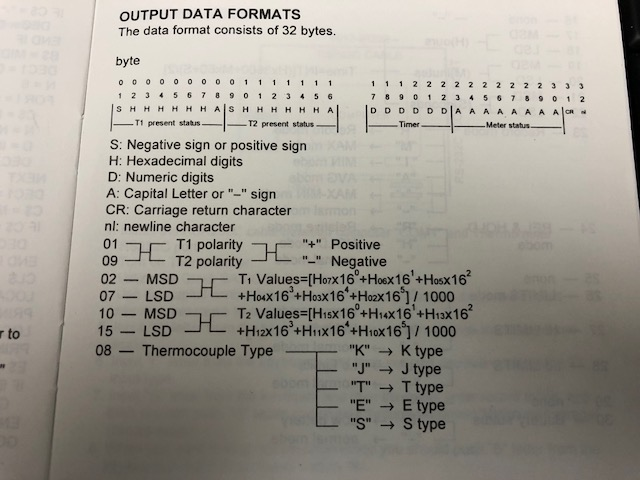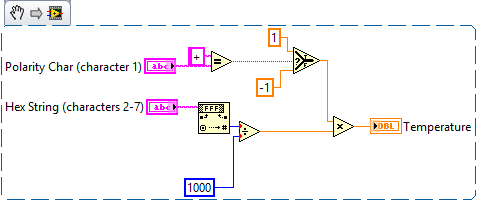# Convert Hex String to Number

## Recommended Posts

I need to convert a string (+0056A4K+005640_000353________) into two temperature readings.

I get the first character is the first temperature reading polarity, and I get the seventh character is the thermocouple type. it's what's happening in the middle that has me stumped.

How do I convert 0056A4 to the temperature. I've attached the image of the directions, but I can't figure them out.

Any help would be greatly appreciated.

BobIf I am reading the directions correctly, you can break this reading up into sections:

(character 1) T1 Polarity = +

(characters 2-7) T1 Value = 0x0056A4 = 22180

(character 8) Thermocouple Type = K

(character 9) T2 Polarity = +

(characters 10-15) T2 Value = 0x005640 = 22080

(character 16) Unknown = _

(character 17-22) Timer =000353

(character 23-30) Meter Status = ________

(character 31) = Carriage Return (part of a new line character)

(character 32) = Line Feed (also part of a new line character)

The calculation shown is to convert a hex value to a decimal value and scale it into an actual temperature (divide by 1000).  Mentioning MSD and LSD is just so you can order the Hex value correctly when converting to decimal.

0x0056A4 = 0 * 16^5 + 0 * 16^4 + 5 * 16^3 + 6 * 16^2 + A * 16^1 + 4 * 16^0 = 0 * 1048576 + 0 * 65536 + 5 * 4096 + 6 * 256 + 10 * 16 + 4 * 1 = 0 + 0 + 20480 + 1536 + 160 + 4 = 22180

Your temperatures are 22.180 and 22.080 (not sure what unit; probably degrees Celsius).

In LabVIEW, you can use code similar to the following to make the conversion:Conversion Example.vi

•1

Thank you... Great explanation....

## Join the conversation

You can post now and register later. If you have an account, sign in now to post with your account.×   Pasted as rich text.   Paste as plain text instead

Only 75 emoji are allowed.

×   Your previous content has been restored.   Clear editor

×   You cannot paste images directly. Upload or insert images from URL.

×
×